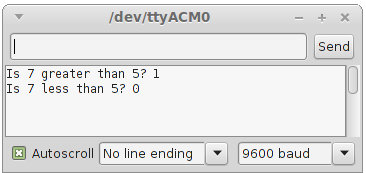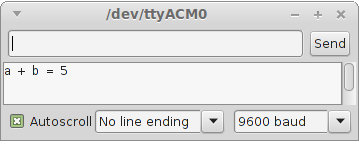Starting Electronics Needs Your Help!
It is that time of the year when we need to pay for web hosting and buy new components and equipment for new tutorials. You can help by making a donation. Contribute to this website by clicking the Donate button. The total will be updated once daily. (You may need to clear your browser cache to see the updates.)Target Amount: \$2000
Amount Raised: \$1579.10

Top Donor: C.C. \$100

X

# Relational Operators

Created on: 26 September 2014 | Updated on: 19 January 2017

Part 5 of the Arduino Programming Course

Relational operators test the relationship between values, for example is the number 7 greater than the number 5; or is the value that a variable holds less than 10.

The result of a comparison of two values by a relational operator will either be true or false, for example is the value contained in a variable equal to 8? The answer will either be true (it does hold the value 8) or false (it does not hold the value 8).

In programming, true is represented by the value 1 and false is represented by the value 0. The example that follows will help to clarify this explanation of relational operators.

## Relational Operator Example

The following sketch uses the greater than (>) relational operator and the less than (<) relational operator to compare two numbers.

```void setup() {
Serial.begin(9600);

Serial.print("Is 7 greater than 5? ");
Serial.println(7 > 5);

Serial.print("Is 7 less than 5? ");
Serial.println(7 < 5);
}

void loop() {
}
```

The output of the above sketch is shown here.The first relational operator tests whether 7 is greater than 5: 7 > 5

7 is greater than 5, so the output from this comparison is true or 1.

The second relational operator tests whether 7 is less than 5: 7 < 5

7 is not less than 5, so the output from the comparison is false or 0.

## Types of Relational Operators

The Arduino programming language has 6 relational operators listed below.

### Greater Than >

We have already seen the greater than relational operator working in the above example. Here is another example using the same operator to compare the contents of two variables.

```int a = 2;
int b = 3;

Serial.println(a > b);
```

The relational operator asks the question "Is a greater than b?".

The result of the comparison will be 0 (false) in this example because the value that variable a contains is not greater than the value that variable b contains.

### Less Than <

Here we use the less than relational operator to compare the contents of the same two variables.

```int a = 2;
int b = 3;

Serial.println(a < b);
```

The relational operator asks the question "Is a less than b?".

The output of this sketch will be 1 (true) because the value that variable a contains is less than the value that variable b contains.

### Greater Than or Equal To >=

This operator acts in the same way as the greater than operator, but will also evaluate to true (1) if the two values being compared are equal to each other.

### Less Than or Equal To <=

This operator will evaluate to true (1) if the value on the left of the operator is less than the value on the right of the operator; or if the two values are equal.

### Equal To ==

The equal to relational operator will only evaluate to true (1) if both values being compared are equal, otherwise it will evaluate to false (0).

It is easy to forget to use two equals signs (==) and use only one equals sign for the equal to relational operator. Using only one equals sign will cause the variable on the left of the operator to be assigned the value of the variable or constant on the right. If the value on the left is a constant, a compile error will occur if a single equals (=) is used instead of a double equals (==).

### Not Equal To !=

The not equal to relational operator will only evaluate to true if the two values being compared to each other are not equal to each other, otherwise it will evaluate to false.

## Relational Operator Sketch

The Arduino sketch below demonstrates relational operators.

```void setup() {
int a = 2;
int b = 3;

Serial.begin(9600);

Serial.print("Is a greater than b? ");
Serial.println(a > b);

Serial.print("Is a less than 25? ");
Serial.println(a < 25);

Serial.print("Is a greater than or equal to b? ");
Serial.println(a >= b);

a = 3;
Serial.print("Is a greater than or equal to b? ");
Serial.println(a >= b);

a = 4;
Serial.print("Is a greater than or equal to b? ");
Serial.println(a >= b);

Serial.print("Is a equal to b? ");
Serial.println(a == b);

b = 4;
Serial.print("Is a equal to b? ");
Serial.println(a == b);

}

void loop() {
}
```

This video shows the output produced from the above sketch after running it.

Can't see the video? View on YouTube →

Copy this sketch and run it on your Arduino. Experiment with relational operators to make sure that you understand them.

### Things to Note in the Sketch

#### Evaluating and Printing an Expression

Something to note in the above sketches is that an expression is evaluated and printed in one statement. The result of the relational operator expression is not first saved to a variable and then printed.

This can also be done with arithmetic operators as shown here:

```void setup() {
int a = 2;
int b = 3;

Serial.begin(9600);

Serial.print("a + b = ");
Serial.println(a + b);
}

void loop() {
}
```

The output of the above sketch is shown here:The expression a + b is both evaluated (to get the value of 5) and printed in the same statement.

#### Confusing = and ==

As already noted, it is easy to forget the second equals sign when using the equal to operator. The sketch below shows what can happen with this mistake.

```void setup() {
int a = 2;
int b = 3;

Serial.begin(9600);

Serial.print("a == b: ");
Serial.println(a == b);

Serial.print("a = b: ");
Serial.println(a = b);
}

void loop() {
}
```

The comparison of a and b in the expression a == b is correct and evaluates to 0 as expected.

If the second equals sign is left off as shown in the second expression a = b, the assignment operator (=) is accidentally used instead of the equal to operator (==).

This causes a to be assigned the value of b which is 3 and then 3 is printed out. A non-zero number will actually evaluate to true as we will see later in this course.